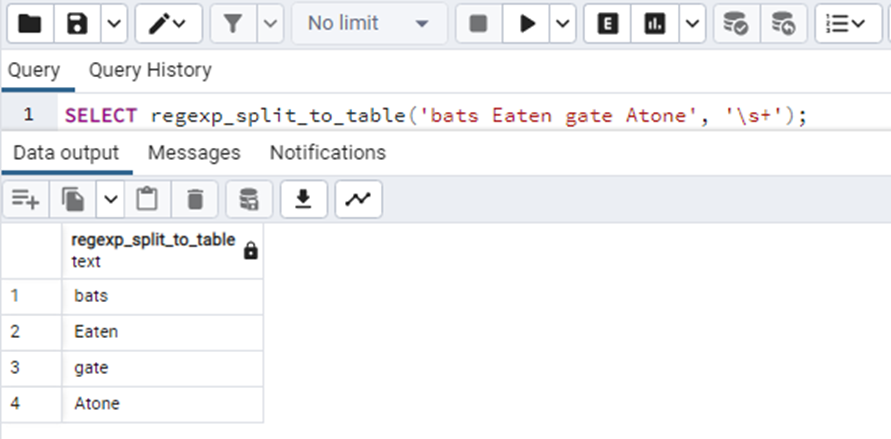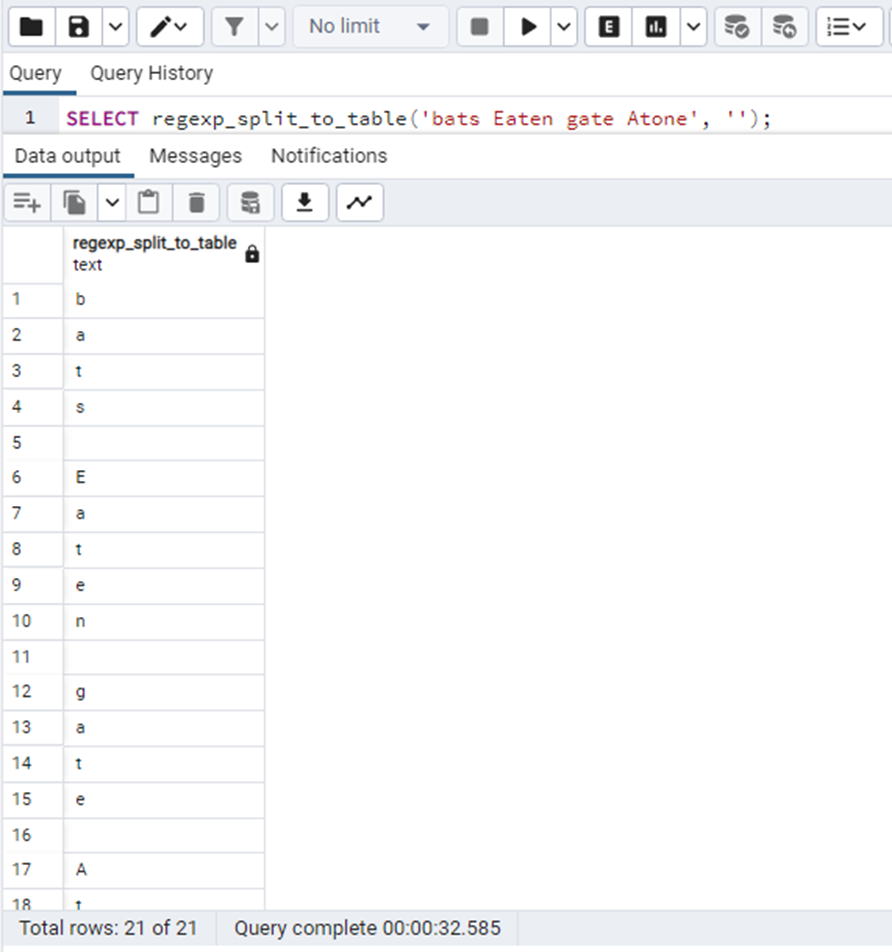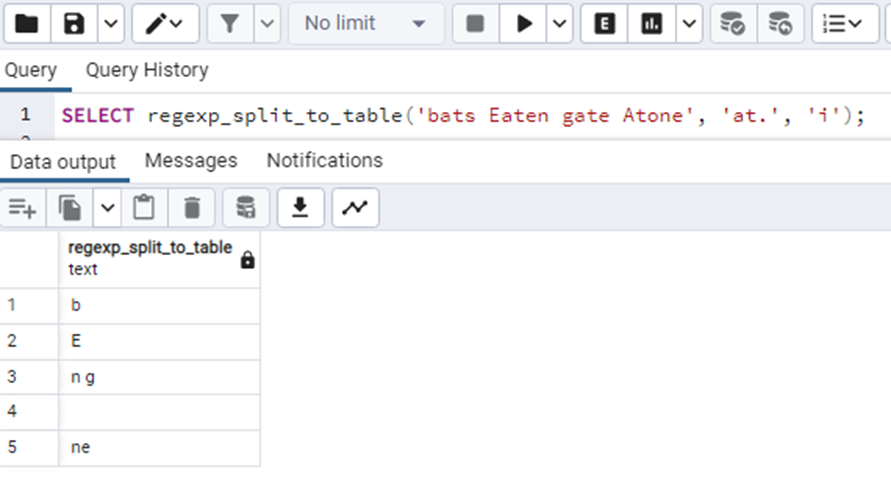##### PostgreSQL regexp_split_to_table() Function

In Postgresql, the regexp_split_to_table() function is used to split the specified string into a table column using the specified POSIX regular expressions. The strings as well as the POSIX regular expression are provided to the function as an argument. This expression will act as a separator for the string elements in the table column. A flag can also be specified in the function. This function works the same as the string_to_table() function.

Let’s get deep into the details of the regexp_split_to_table() function.

## PostgreSQL regexp_split_to_table() Function

As discussed earlier, the regexp_split_to_table() function splits the given string into a table column placing the regular expressions as separators. There also exists a third argument, which is completely optional, that can control some specific behavior of the function. The function returns a table. Let's have a look at the basic syntax of the function:

`regexp_split_to_table(String,   Regexp[, Flag]);`

In the above syntax:

● The String is the main string that needs to be split.

● The second argument, Regexp, is the POSIX reg expression that will act as a separator.

● The third argument is the flag that can slightly mold the functionality of the function. For example, the ”i” flag will consider the sensitive matching.

The function returns a table that contains the string split in the columns with the regular expressions as separators. Below are some of the important things about the value of Regex:

● If the Regex is NULL the function will also return NULL.

● If the value of Regex is an empty string, the function will simply return all the characters of the original string.

Let’s move on to the examples of the regexp_split_to_table() function to understand it better.

Example

Consider the following query as an example:

`SELECT regexp_split_to_table('bats   Eaten gate Atone', '\s+');`

In this query, the string, 'bats Eaten gate Atone', will be separated by whitespaces as the regular expression is ”\s+” which gives the white spacing between the string elements and separates them in column rows. The output of the query is:Consider the case where the Regex is an empty string. The query is as follows:

`SELECT regexp_split_to_table('bats   Eaten gate Atone', '');`

The output of this query will separate all the characters of the string in the columns of a table like this:Now consider using the optional flags in the query. In the below query, we will be using the “i” flag.

`SELECT regexp_split_to_table('bats   Eaten gate Atone', 'at.', 'i');`

The “i” flag will match the regex for the case-insensitive characters in the string and then accordingly return the value like this:In the above output, the” i” flag has found “at” in the string and its next character(represented by “.”) and separated them in the columns of a table.

This was all about the regexp_split_to_table() function.

## Conclusion

The regexp_split_to_table() function takes the string, the regexp, and the flag(if any) as arguments and returns a table column that contains the string elements/characters with the Regexp as separators. In this article, we have learned about the regexp_split_to_table() function in PostgreSQL. This function works the same as the string_to_table() function does.